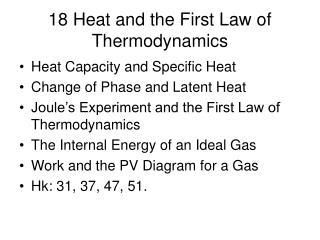DownloadDownload Presentation18 Heat and the First Law of Thermodynamics

# 18 Heat and the First Law of Thermodynamics

Download Presentation## 18 Heat and the First Law of Thermodynamics

- - - - - - - - - - - - - - - - - - - - - - - - - - - E N D - - - - - - - - - - - - - - - - - - - - - - - - - - -
##### Presentation Transcript

1. 18 Heat and the First Law of Thermodynamics • Heat Capacity and Specific Heat • Change of Phase and Latent Heat • Joule’s Experiment and the First Law of Thermodynamics • The Internal Energy of an Ideal Gas • Work and the PV Diagram for a Gas • Hk: 31, 37, 47, 51.

2. Heat & Internal Energy • heat is energy that flows due to temperature differences. SI Unit: joule • internal energy is the total energy of an object in its center of mass reference frame

3. 0 Specific Heat Capacity • The amount of heat needed to raise 1kg by 1°C • c = Q/mDT SI Unit: J/kg·°C • m = mass receiving (or emitting) heat • DT = temperature change • Q = mcDT • /

4. 0 Example: Calorimetry Example: 300J of heat are added to 100grams of a substance. The temperature rises by 2.20 degrees C. The specific heat of the substance is:

5. 0 Other Heat & Energy Units:

6. 0 Example Conversion A Gas Range is quoted (incorrectly) as “12,000 Btu”. The equivalent power for an Electric Range in watts is:

7. Solid/Liquid Transformation Melting Heat in KE breaks bonds Molecules move Liquid forms Freezing Heat out Molecules lose KE Settle in one place Solid forms

8. Energy in Phase Change Energy added: solid  liquid  gas energy released: gas  liquid  solid 8

9. Liquid/Gas Transformations Evaporation Highest KE molecules escape liquid from surface Evaporative cooling, e.g. sweat evaporates cooling your body Boiling Highest KE molecules form gas bubbles throughout Hot gas bubbles rise and escape at surface Liquid has passed heat out, e.g. simmering at constant temperature

10. Latent Heat • Energy needed to melt or vaporize 1kg of a substance. • Latent Heat, L = Q/m. • Q = mL. • Example: 2.5kg of ice at 0.0°C melts into 2.5kg of water at 0.0°C.

11. 0 Joule’s Experiment confirmed the mechanical equivalent of heat.

12. 0 1st Law Thermodynamics

13. 18-4 Internal Energy of Ideal Gas • E-internal is proportional to the absolute temperature • internal energy is in the kinetic energy of the molecules of the gas • /

14. Work and the PV Diagram

15. Gas Processes • Isobaric = constant pressure • Isometric (Isochoric) = constant volume • Isothermal = constant temperature • Ex.

16. 0 Summary: • specific heat is material dependent. • latent heats; objects change phase at constant temperature. • calorimetry: science of heat measurements • 1st law of thermo. is energy conservation • mechanical equivalent of heat • Pressure x volume = work

17. 0

18. 0 Example: Human vs. Gasoline Engine Lance Armstrong: 460W V8: 200hp Power Ratio = 200hp/(460/746)hp = 332 times more Energy Consumption (6h = 21,600s): Lance: (460W)(21,600) = 9.9 MJ = 2370 Cal V8: (332 times more) = 3.3 GJ = 788,000 Cal

19. 0 Which of the following is a unit of heat? • N/m • N/m2 • N·s • N·m

20. 0 Heat is added to a system and the change in internal energy of the system is 1/3 of the heat added. Which of the following is true? • Work on system is + • Work on system is – • Work on system is 0

21. 0 When not colliding, molecules of an ideal gas • Attract • Repel • Neither attract or repel

22. 0 Molecules in an ideal gas • Never collide • Sometimes collide

23. 18-5Work and PV Diagrams

24. Work and PV • [PV] = (N/m2)(m3)= joule = energy • Work done by an expanding gas: • Work done by gas during isothermal expansion:

25. Internal Energy of an Ideal Gas • is proportional to temperature of gas • internal energy is in the kinetic energy of molecules of the gas